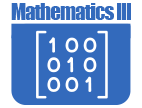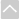# Math - Secondary Math IIIOER Curriculum

These Open Educational Resources are comprehensive and coherent curricular materials that may be used to teach a course or grade level.

The Math Vision Project
• Introduction to the Materials (Math 3)
Introduction to the Materials in the Mathematics Three of the The MVP classroom experience begins by confronting students with an engaging task and then invites them to grapple with solving it. As students ideas emerge, take form, and are shared, the teacher orchestrates the student discussions and explorations towards a focused mathematical goal. As conjectures are made and explored, they evolve into mathematical concepts that the community of learners begins to embrace as effective strategies for analyzing and solving problems.
• Module 1: Functions and Their Inverses - Student Edition (Math 3)
The Mathematics Vision Project, Secondary Math Three Module 1, Functions and Their Inverses, reviews the features of linear, exponential, and quadratic functions and general inverse relationships. The idea that the inputs and outputs are reversed in inverse functions is reinforced in the module using tables, graphs, equations, and story context.
• Module 1: Functions and Their Inverses - Teacher Edition (Math 3)
The Mathematics Vision Project, Secondary Math Three Module 1, Functions and Their Inverses, reviews the features of linear, exponential, and quadratic functions and general inverse relationships. The idea that the inputs and outputs are reversed in inverse functions is reinforced in the module using tables, graphs, equations, and story context.
• Module 2: Logarithmic Functions - Student Edition (Math 3)
The Mathematics Vision Project, Secondary Math Three Module 2, Logarithmic Functions, picks up where Module 1 leaves off. Students begin to understand logarithms by drawing upon their experiences with inverses and exponential functions to evaluate, approximate, and order logarithmic expressions such log2 8 and log2 20.
• Module 2: Logarithmic Functions - Teacher Edition (Math 3)
The Mathematics Vision Project, Secondary Math Three Module 2, Logarithmic Functions, picks up where Module 1 leaves off. Students begin to understand logarithms by drawing upon their experiences with inverses and exponential functions to evaluate, approximate, and order logarithmic expressions such log2 8 and log2 20.
• Module 3: Polynomial Functions - Student Edition (Math 3)
The Mathematics Vision Project, Secondary Math Three Module 3, Polynomial Functions, begins with a task that links linear, quadratic, and cubic functions together by highlighting the rates of change of each function type and using a story context to show that a linear function is the sum of a constant, a quadratic function is the accumulation or sum of a linear function, and a cubic function is the sum of a quadratic function.
• Module 3: Polynomial Functions - Teacher Edition (Math 3)
The Mathematics Vision Project, Secondary Math Three Module 3, Polynomial Functions, begins with a task that links linear, quadratic, and cubic functions together by highlighting the rates of change of each function type and using a story context to show that a linear function is the sum of a constant, a quadratic function is the accumulation or sum of a linear function, and a cubic function is the sum of a quadratic function.
• Module 4: Rational Expressions and Functions - Student Edition (Math 3)
The Mathematics Vision Project, Secondary Math Three Module 4, Rational Expressions and Functions, students work with the fractions that are ratios of polynomials, rational expressions and functions.
• Module 4: Rational Expressions and Functions - Teacher Edition (Math 3)
The Mathematics Vision Project, Secondary Math Three Module 4, Rational Expressions and Functions, students work with the fractions that are ratios of polynomials, rational expressions and functions.
• Module 5: Modeling with Geometry - Student Edition (Math 3)
The Mathematics Vision Project, Secondary Math Three Module 5, Geometric Modeling, begins with students visualizing two-dimensional cross sections of three-dimensional objects and solids of rotation. They learn to approximate the volume of an irregular solid by decomposing it into cylinders, frustrums, and cones with volumes that can be easily calculated.
• Module 5: Modeling with Geometry - Teacher Edition (Math 3)
The Mathematics Vision Project, Secondary Math Three Module 5, Geometric Modeling, begins with students visualizing two-dimensional cross sections of three-dimensional objects and solids of rotation. They learn to approximate the volume of an irregular solid by decomposing it into cylinders, frustrums, and cones with volumes that can be easily calculated.
• Module 6: Modeling Periodic Behavior - Student Edition (Math 3)
The Mathematics Vision Project, Secondary Math Three Module 6, Modeling Periodic Behavior. In this module students use a Ferris wheel as a context for constructing conceptual understanding of circular trigonometry. They begin by calculating heights on the Ferris wheel, progress to calculating the heights at a given time on the Ferris wheel, and then, graphing the heights to show a sine function.
• Module 6: Modeling Periodic Behavior - Teacher Edition (Math 3)
The Mathematics Vision Project, Secondary Math Three Module 6, Modeling Periodic Behavior. In this module students use a Ferris wheel as a context for constructing conceptual understanding of circular trigonometry. They begin by calculating heights on the Ferris wheel, progress to calculating the heights at a given time on the Ferris wheel, and then, graphing the heights to show a sine function.
• Module 7: Trig. Functions, Equations & Identities - Student Edition (Math 3)
The Mathematics Vision Project, Secondary Math Three Module 7, Trigonometric Functions, Equations, and Identities, students work with more trigonometric graphs, beginning with the familiar Ferris wheel context.
• Module 7: Trig. Functions, Equations & Identities - Teacher Edition (Math 3)
The Mathematics Vision Project, Secondary Math Three Module 7, Trigonometric Functions, Equations, and Identities, students work with more trigonometric graphs, beginning with the familiar Ferris wheel context.
• Module 8: Modeling With Functions - Student Edition (Math 3)
The Mathematics Vision Project, Secondary Math Three Module 8, Modeling with Functions, focuses on a big idea of functions: Functions can be combined together (using basic operations or composed) to make new functions, usually retaining some of the features of both functions.
• Module 8: Modeling With Functions - Teacher Edition (Math 3)
The Mathematics Vision Project, Secondary Math Three Module 8, Modeling with Functions, focuses on a big idea of functions: Functions can be combined together (using basic operations or composed) to make new functions, usually retaining some of the features of both functions.
• Module 9: Statistics - Student Edition (Math 3)
The Mathematics Vision Project, Secondary Math Three Module 9, Statistics, begins with a learning cycle where students construct the concept of normal distributions, understanding the effect of modifying either the mean or standard deviation.
• Module 9: Statistics - Teacher Edition (Math 3)
The Mathematics Vision Project, Secondary Math Three Module 9, Statistics, begins with a learning cycle where students construct the concept of normal distributions, understanding the effect of modifying either the mean or standard deviation.
•Engage NY

• High School Mathematics
In order to assist educators with the implementation of the Common Core, the New York State Education Department provides curricular modules in Pre-K-Grade 12 English Language Arts and Mathematics that schools and districts can adopt or adapt for local purposes.Georgia Standards of Excellence Curriculum Frameworks
• Georgia Standards of Excellence Mathematics
GeorgiaStandards.Org (GSO) is a free, public website providing information and resources necessary to help meet the educational needs of students. The goal of this web site is to provide information that will enhance and support teaching and learning of Georgia standards.The Online Core Resource pages are a collaborative project between the Utah State Board of Education and the Utah Education Network. If you would like to recommend a high quality resource, contact Trish French (Elementary) or Lindsey Henderson (Secondary). If you find inaccuracies or broken links contact resources@uen.org.